• 图像的亚像素移动，分为垂直和水平方向的分向量 注释详细 生成移动后图片结果保存在work space
• 三、图像像素级标注 1、Load选择一张图像设置ROILabels 2、标注结束，保存文件ExportLables -->to files, 3、保存文件说明 保存路径下有两个文件，在文件夹中打开Label_1.png会是一张黑色的图像，要在...
目录
一、Matlab 版本：2020a
二、启动 Image Labeler
三、图像像素级标注
2、标注结束，保存文件 Export Lables -->to files,
3、保存文件说明
4、标注保存为csv文件
5、查看标注后的图像
6、最终的效果

一、Matlab 版本：2020a

二、启动 Image Labeler
matlab 命令行输入 imageLabeler，回车，打开界面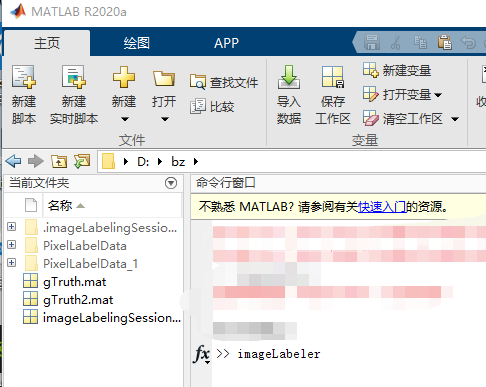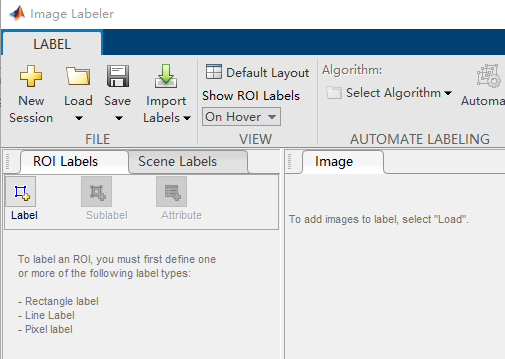三、图像像素级标注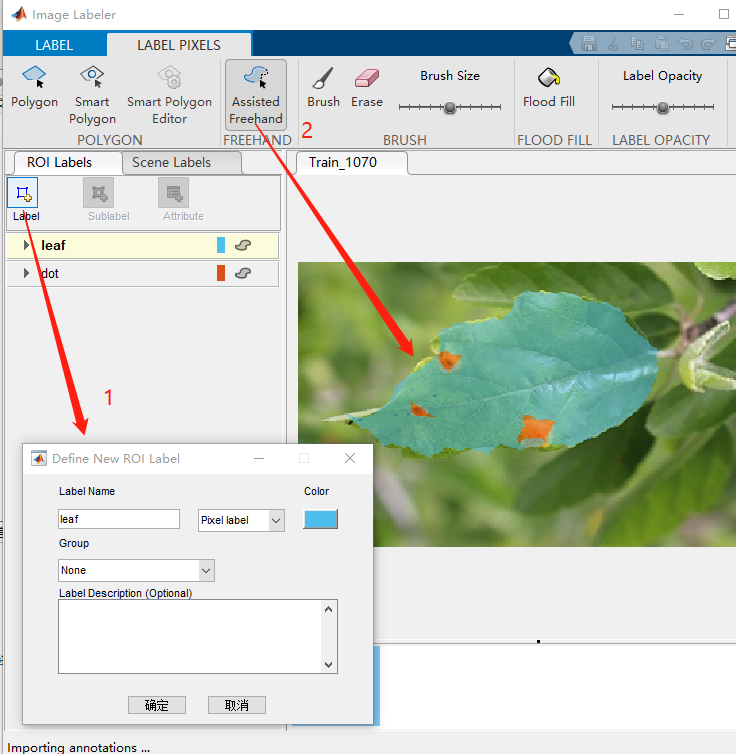2、标注结束，保存文件 Export Lables -->to files,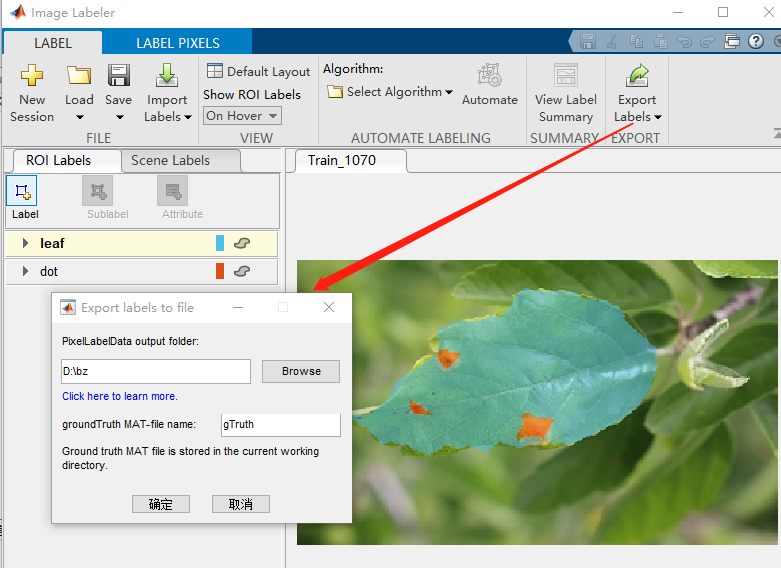3、保存文件说明
保存路径下有两个文件，在文件夹中打开Label_1.png 会是一张黑色的图像，要在matlab 中打开。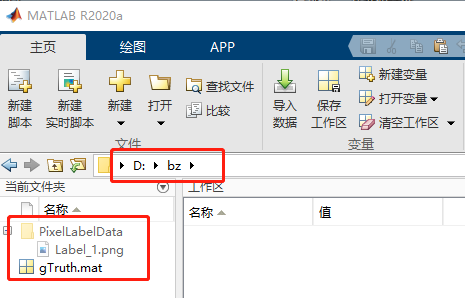4、标注保存为csv文件
双击Label.png 图像，弹出对话框点击完成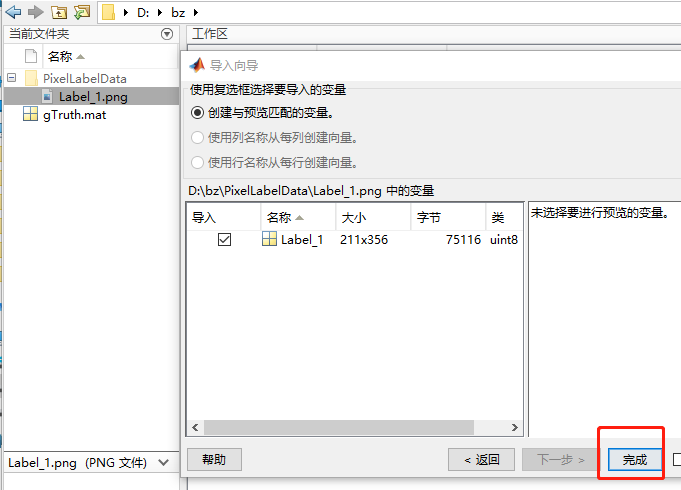在工作去会显示Lable_1 和图像的尺寸，标注（背景为0 ，分类标注1,2，.....）
双击打开，再ctrl+A全选，复制到 excel文件中，另存为csv就可以了。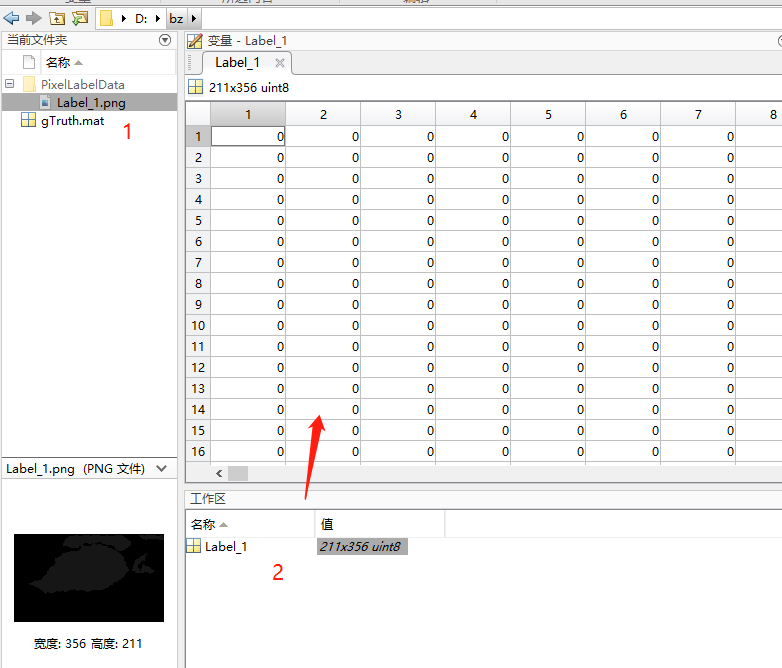5、查看标注后的图像
def csv2Img(csvFile,colorKey=None,filePath=None):
'''
将标记的csv转换成图像
--------
:param csvFile: 标注的文件路径
:param colorKey: 类对应的RGB值
:param filePath: 图片保存路径
:return: None

'''
if colorKey==None:
colorKey = {1: [0, 0, 255], 2: [0, 255, 0], 3: [255, 0, 0], 4: [128, 128, 128]}
# Colour key
ground_truth = np.loadtxt(open(csvFile, "rb"), delimiter=',', skiprows=0)
csv_shape = ground_truth.shape
imgPlace = np.zeros(shape=(csv_shape, csv_shape, 3))
for x in range(0, csv_shape):
for y in range(0, csv_shape):
if ground_truth[y][x]==1:
print()
color = colorKey.get(ground_truth[y][x])
imgPlace[y, x, :] = color

cv2.imshow(filePath, imgPlace)
cv2.imwrite(filePath, imgPlace)
cv2.waitKey(0)

print("csv to img finish 。。。。path:"+filePath)
# 根据自己的类，设置colourkey 值
colourkey = {1: [234, 217, 153], 2: [0, 242, 255], 3:[128, 128, 128]}
csv2Img("imge_lable.csv", colorKey=colourkey,filePath="test.png")
6、最终的效果
原始图像：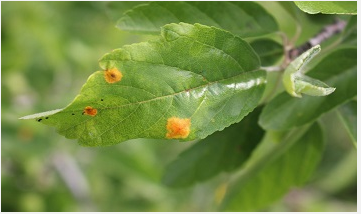像素标注的图像：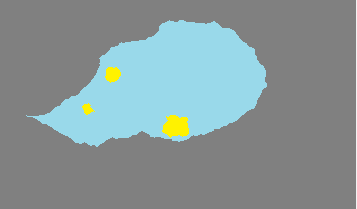展开全文• MATLAB图片点的像素坐标，可以精准的查看图片上每一个点的坐标，很精确，达到0.0001个像素
• matlab中导入PNG图像后，利用size()函数获得总的像素点，并且利用RGB范围来判断五种颜色的像素点各自的个数。
• 图片像素提取。 有童鞋需要把图片变成大的像素点然后做手工送给老师，同样可以应用于钉子画之类的送男票女票，彩色图片原理相同。 原理：图像灰度化 -> 图像二值化 -> 灰度阈值处理 -> 按照一定长度像素...
图片像素提取。
有童鞋需要把图片变成大的像素点然后做手工送给老师，同样可以应用于钉子画之类的送男票女票，彩色图片原理相同。
原理：图像灰度化 -> 图像二值化 -> 灰度阈值处理 -> 按照一定长度像素的小格子划分 -> 小格子黑色大于一定比例设为黑色否则白色
原图

不带划线的图片

带划线的图片

参数：
imagepath = '7d270e2e121daeabafe0513124bc30a.jpg';   %待处理图片 subimgpath = '76bbefdcd560710cfe47045257dec51.jpg'   %可以选择填充子图 sz =3;         %设置分割块的大小 thresh = 125/255;       %灰度阈值 blackthresh = 0.6;  %小格子黑色大于这个值小格子为黑 hasline = 1;   %设置分割线条为1 其他值为没有 linecolor = 0;   %设置分割线条颜色 pixpath = 'a.txt';   %设置最后像素矩阵的路径

imagepath = '7d270e2e121daeabafe0513124bc30a.jpg'; %待处理图片
subimgpath = '76bbefdcd560710cfe47045257dec51.jpg' %可以选择填充子图
sz =3;         %设置块的大小
thresh = 125/255;       %灰度阈值
blackthresh = 0.6;  %小格子黑色大于这个值小格子为黑
hasline = 1; %设置分割线条为1 其他值为没有
linecolor = 0; %设置分割线条颜色
pixpath = 'a.txt'; %设置最后像素矩阵的路径

%figure();%图片太大截图
%A=A(1:360,600:961);
%imshow(I2)
B = rgb2gray(B);
IB=imresize(B,[sz+1,sz+1]);
%thresh = graythresh(A);     %自动确定二值化阈值
I2 = im2bw(A,thresh); %对图像二值化
I2=imresize(I2,[600,600]);%太大的图像需要缩小
figure();
imshow(A);    %显示二值化之前的图片
title('原图');
imshow(I2);    %显示二值化之后的图片
title('二值化');

%I2=1*ones(16)
I2 = uint8(I2);
rs = size(I2, 1); cs = size(I2, 2);%取图像的行列数
ch = sz; cw = sz;

numr = rs/sz;
numc = cs/sz;
tagm = zeros(numr,numc);
t1 = (0:numr-1)*ch+1 ;
t3 = (0:numc-1)*cw+1;
figure;
for i = 1 : numr-1
for j = 1 : numc-1
if hasline == 1
I2(t1(i),:,:)=linecolor;  %设置分割线条
I2(:,t3(j),:)=linecolor;
end
if sum(sum(I2(t1(i)+1:t1(i)+sz,t3(j)+1:t3(j)+sz)==0))/(sz*sz)>blackthresh
I2(t1(i):t1(i)+sz,t3(j):t3(j)+sz)=0;
tagm(i,j)=1;
else
I2(t1(i):t1(i)+sz,t3(j):t3(j)+sz)=255;
tagm(i,j)=0;
end
end
end
imshow(I2);
fid=fopen(pixpath,'wt');%改为你自己文件的位置
[m,n]=size(tagm);
for i=1:m
for j=1:n
if j==n
fprintf(fid,'%g\n',tagm(i,j));
else
fprintf(fid,'%g\t',tagm(i,j));
end
end
end
fclose(fid);



展开全文• 当我们读取已经保存好的图片（jpg BMP等格式）时，用image imshow等函数显示经常会有失真效果，按照以下步骤可以使得显示的图片效果更清晰： set(0,'units','pixes'); %获取图片的屏幕的分辨率 screensize0 = get...
当我们读取已经保存好的图片（jpg BMP等格式）时，用image imshow等函数显示经常会有失真效果，按照以下步骤可以使得显示的图片效果更清晰：
set(0,'units','pixes');
%获取图片的屏幕的分辨率 screensize0 = get(0,'MonitorPosition');    scrwidth = screensize0 (3); %%屏幕宽度     screight = screensize0 (4);    %%屏幕高度
rowscolsm= size(M);%%最后一个是rgb,前两个是分辨率的宽高
maxval=max([rowscolsm(2)/scrwidth rowscolsm(1)/screight]);
M=imresize(M,[rowscolsm(1)/maxval  rowscolsm(1)/maxval],'bilinear'); %%按照 屏幕分辨率和图片本身分辨率进行压缩，压缩算法用的是双线性差值，这个差值算法可以在以下三个里面选：
'nearest'最近邻插值（默认）
'bilinear'双线性插值
'bicubic'双三次插值
试了下效果，第一种算法效果最差，后面两个效果差不多
image(M);

展开全文• 利用Matlab读取图片各个像素的RGB颜色值，然后在Excel中将各像素RGB颜色对应地填充到单元格中。
• 使用MATLAB任意修改图片像素大小 介绍 使用MATLAB把一个任意像素大小的图片修改成想要的像素大小（把一个像素为500* 500的图片修改成像素为200* 200大小的图片），其实很简单一个imresize()函数就搞定，具体效果如下...
使用MATLAB任意修改图片像素大小
介绍
使用MATLAB把一个任意像素大小的图片修改成想要的像素大小（把一个像素为500* 500的图片修改成像素为200* 200大小的图片），其实很简单一个imresize()函数就搞定，具体效果如下所示。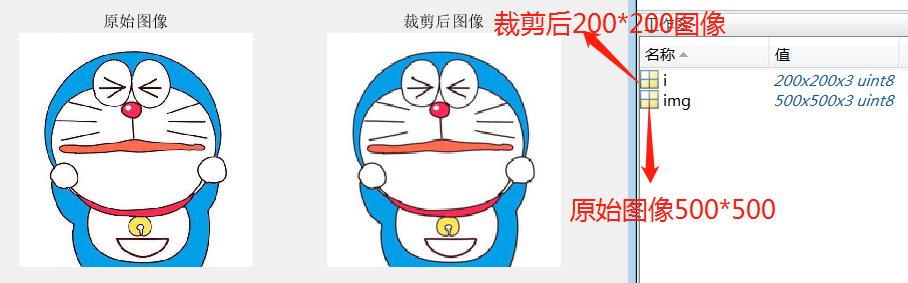从上图可以看出图像大小虽然没有变化但是图像的质量确稍微发生了一些变化，如上图中工作区显示img大小为500500修改后i为一个200200大小的图片。
代码
clc;
clear;
i = imresize(img,[200,200]);  %imresize函数修改图片像素尺寸[]中的内容是想要的像素的长和宽
figure(1)
subplot(1,2,1);imshow(img),title('原始图像');   %显示原始图像500*500*3
%subplot(m,n,p)中的参数含义分别是，行数为m，列数为n，后面的第p个图像
subplot(1,2,2);imshow(i),title('裁剪后图像');   %显示裁剪后图像200*200*3

到最后我们想要的结果就出来了，就不用那些付费操作才能修改图片像素大小了哈哈。
展开全文• 执行快速准确的亚像素边缘检测，基于改进的zernike矩方法，有需要的可以下载~~
• Matlab如何用鼠标获取图像像素值和坐标-test.fig 如题 我是在一个GUI里，有两个axe，分别用来读取两个图片 我想要实现的是：图片在axe显示后，当鼠标在图片上移动，或者点击，可以得到图片像素值和其在axe中...
• Matlab如何用鼠标获取图像像素值和坐标-test.m 如题 我是在一个GUI里，有两个axe，分别用来读取两个图片 我想要实现的是：图片在axe显示后，当鼠标在图片上移动，或者点击，可以得到图片像素值和其在axe中的...
• 图像的代数运算是指将两幅或多幅图像通过对应像素之间的加、减、乘、除运算得到输出图像的方法。 在MATLAB图像数据类型是unit8，当进行代数运算时有可能产生属性溢出，所以应当在进行图像代数运算之前首先将数据...
• 可测试像素点的matlab程序 % 主函数 clear global c; for k=1:6 clear global a; clear global i; clear n fn; global a; global i; global c; global p; p = 1; i = 1; % a(:,:)=[]; % b(:,:,:)=[]; n=...
• A=imread("C:\Users\HP\Desktop\kodim22.png"); % get the histogram [Y,X]=size(A); % Y Row , X column grayvalue=unique(A); imginfo=[]; for i=1:length(grayvalue) [ANSy,ANSx]=find(A==grayvalue(i))...
• 实现MATLAB图片批量归一化，去掉图像中面积(像素点总数）小于50的区域之后，将图像归一化为256*256的图片
• 它利用像素之间特征的相似性将像素分组,用少量的超像素代替大量的像素来表达图片特征,很大程度上降低了图像后处理的复杂度，所以通常作为分割算法的预处理步骤。已经广泛用于图像分割、姿势估计、目标跟踪、目标识别...
• %图像相邻像素相关性 %随机选取图像A中相邻的N个像素点，分别计算在水平，垂直，正对角和反对角方向上的相关系数 %注意是算密文图像 R G B分量分开算 function r=ImCoef(A,N) A=double(A);[m,n]=size(A);r=zeros(1,...
• 像素级融合matlab代码，Brovery变换融合也被称为彩色标准变换融合，它是将多光谱波段颜色（红、绿、蓝）归一化，将高分辨率影像与多光谱各波段（通常取三波段）相成完成融合。融合算法非常简单。
• 统计二值化之后，各个黑白图像中0、1 的像素点数目 使用折线图的方式，展示出统计的结果 首先进行输入文件夹 与输出目标文件夹的路径定义： input_path = 'E:\test1\';%输入图片文件夹路径 output_path = 'E:\...
• matlab 实现基于互相关的亚像素图像匹配配准的程序，亲测有用
• ## MATLAB图像处理之图像的像素矩阵

万次阅读 多人点赞 2018-10-02 17:47:40
首先我们用MATLAB打开一张图像可以发现在工作区中就会多一个变量，这个变量是一个三维数组，第一维代表图像的X坐标，第二维代表图像的Y坐标，第三维代表R、G、B，第三维中的数字1代表R，数字2代表G，数字3代表B。...
• 这是Elsevier发表于《Accurate Subpixel Edge Location Based on Partial Area Effect》一文中详述的亚像素边缘检测方法的Matlab源码http://www.sciencedirect.com/science/article/pii/S0262885612001850 主文件夹...
• 获取任意图像位置的像素值 根据坐标获取像素值 P=impixel(im,r,c) r:行坐标 c:纵坐标 P：返回的RGB值 im=imread('C:\Users\14372\Desktop\Music_pictures\图片\梦.png'); r=[40 60 80]; c=[100 120 140]; figure; ...
• 它将输入图像映射为输出图像，输出图像每个像素点的灰度值仅由对应的输入像素点的灰度值决定，运算结果不会改变图像像素点之间的空间关系。简而言之，点运算仅仅改变了图像像素点的灰度值。 其运算的数学关系式为...
• 了解了基本的图像文件的读取处理操作之后，我们就要只是如何具体处理图像，让它变成我们想要的图片 点运算又称为对比度增强、对比度拉伸或灰度变换，是一种通过图像中的每一个像素值(即像素点上的灰度值)进行运算的......

# matlab图像像素matlab 订阅